Choose Language Hide Translation BarStaffJoined:

Jun 9, 2012

Lookup tables in JMP

A few days ago, I showed a customer how she could use lookup tables in JMP, and I thought it would be a good idea to share this with everyone.

Those of you who have used lookup tables elsewhere already know how handy they can be. For those who have never used one, let’s first look at a simple example: assigning a letter grade based on a numeric grade.

Suppose we have the following correspondence between numeric and letter grades:

E: x < 60

D: 60 <= x < 70

C: 70 <= x < 80

B: 80 <= x < 90

A: 90 <= x

We’d like to automatically assign letter grades to the numeric grades below — but how?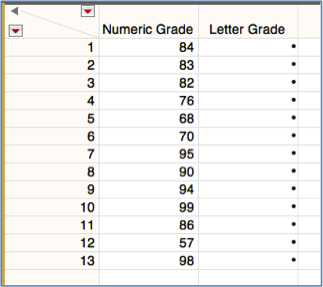The most common way is to use a formula column with an IF clause. Statements are evaluated in the order encountered, and once a statement evaluates to “True,” execution stops — so the clause must be constructed with this in mind: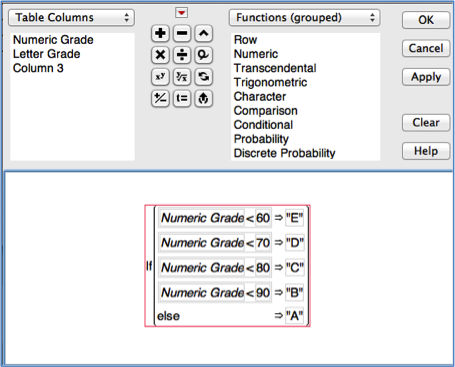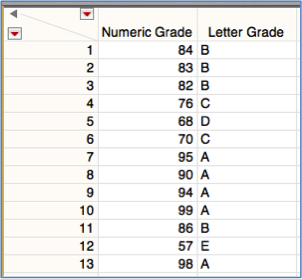This technique works perfectly well, but unfortunately, it can become cumbersome in certain instances:

• What happens if we need to consider a list of many values, not just a few? Writing a long IF clause is tedious and error-prone.
• What if the list of values is not known in advance but determined during run-time? We can handle this by using JSL to write code dynamically, but most people prefer to avoid this if they can.

Fortunately, we can tackle cases like these with the help of a powerful matrix function: Loc Sorted().

How does Loc Sorted() work?

• Loc Sorted(x, y) takes as inputs a matrix x, sorted from low to high, and y, which can be either of matrix or scalar type.
• Loc Sorted() returns the index (or indices, if y is a matrix) of the last value in x that is less than or equal to y.

For example, running this code gives a result of , because 14 is greater than or equal to the number stored in the 3rd position of the x matrix, but less than the number stored in the 4th position of the x matrix:

x = [0, 5,10,15,20,25];

y = 14;

show(loc sorted (x, y));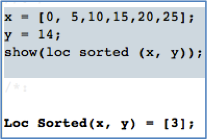Similarly, running this code gives a result of [4,6,6,1]:

x = [0, 5,10,15,20,25];

y = [17, 25, 50, 3];

show(loc sorted (x, y));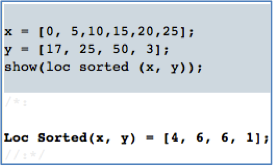Note that the minimum of the x matrix should be the lowest value of y you expect to encounter, because 1 is returned for any y value that is less than all of the x values: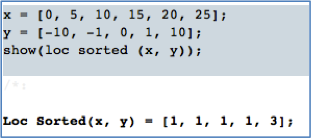In the letter grade example, we can use loc sorted() to pick the grade from list {“E”, “D”, “C”, “B”, “A”}: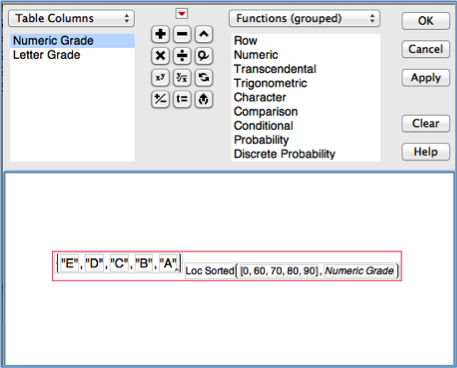This is great if we’ve got only a few categories to consider. But what happens when we have a whole table full of options, or need to not merely look up a single value, but look up several different values and use them together?

Fortunately, this is easy to do. All we need is an extra table to hold the information. Computing tax from a tax table is a classic (and timely) example of such a case.

*** Warning: I am not a tax professional. I do not play one on TV. Please obtain your 2013 tax tables from the IRS.

In today’s example, which is for illustrative purposes only, should not be construed as tax advice, and is not from a tax professional, we will use the table below, which I made from information I found online.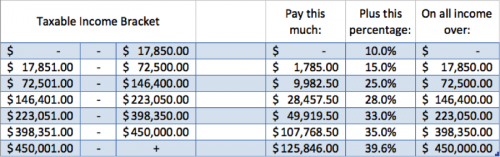Our goal is to compute the tax owed, given the table above and the amount of income being taxed.

First, we need to place this information into a data table (notice that we will lower the contents of the first column by \$1, because of the way Loc Sorted () works.) To follow along with the example, use the following names for the table and columns, or download the example from the JMP File Exchange (download requires free SAS login).

Table name: MarriedFilingJointlyTable

Column names:

• Cuts (income cutoff for each of the tax brackets)
• PreTax (sum of tax owed on income in all lower brackets)
• MargRate (tax rate on last “block” of income)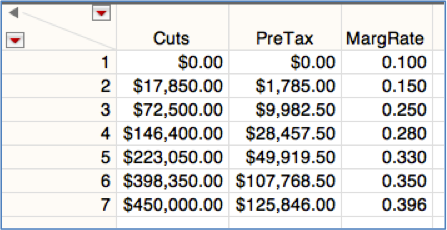We want to use the table above to determine the tax for the taxable incomes in the following table, named MarriedFilingJointlyTable: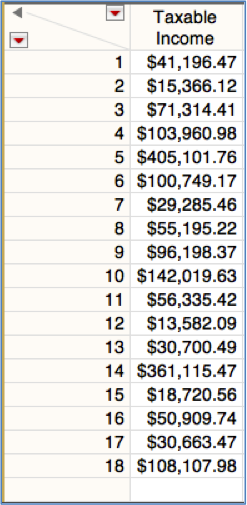Armed with Loc Sorted() and the two tables above, we’re ready to begin.

In the income table, we add a column by selecting Cols > New Column…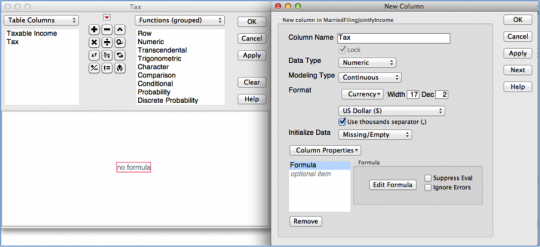We enter “Tax” as the Column Name and select “Currency” as the Format.

We then select “Formula” from the Column Properties drop-down, at which point the Formula Editor appears.

Did you know that we can actually enter a program into a column formula? That is what we will do here — and even though our script is only three lines, the easiest and least error-prone way to do this is by copying and pasting from a script window.

So, open up a script window (File > New > New Script) and type the following: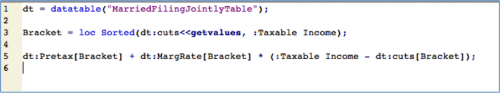Line 1 points the variable dt to the tax table:

• Whenever we want to use a column from the tax table — rather than the income table, where the formula actually will reside — we need to preface that column name with dt.

Line 3 determines which row of the tax table we will use for a given amount of income:

• We call Loc Sorted() using all of the values from the cuts column in the tax table as x, and the value in the current row of the Taxable Income table as y.
• We store the result of this call as Bracket, which we will use to find the row we need in the tax table.
• For example, for the first income value, because \$ 41,196.47 is between the cutoffs contained in rows 2 and 3 of the tax table, Bracket equals 2, so we will end up using row 2 in the tax table to compute the tax.

Line 5 computes the tax. Using the first income value of \$41,196.47 as an example:

• dt:PreTax[Bracket] is the value stored in the appropriate row of the PreTax column of the tax table. For the first income value listed, this is the sum of all taxes on income below the cutoff closest to, but not exceeding, \$41,196.47. (In this case, it is \$1,785.)

To this amount, we must add the product of:

• dt:MargRate[Bracket], the marginal tax rate in the highest bracket at which \$ 41,196.47 is taxed (In this case, it is 0.150), and
• :Taxable Income – dt:cuts[Bracket], which is the amount of income taxed at this rate (In this case, it is \$41,196.47 - \$17,850.)

Once we’ve entered the script into the script window, we select all of it, copy it and paste it into the red box in the formula editor (which until now has contained nothing):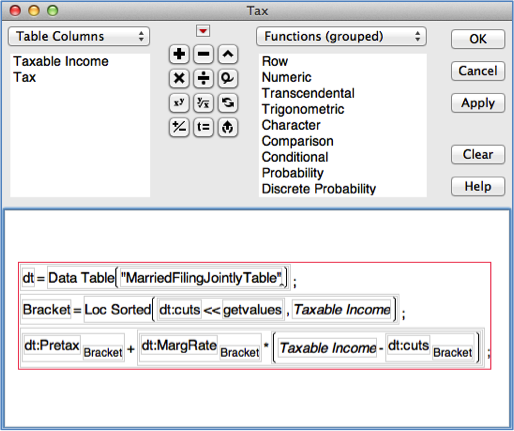After pressing “OK” to close the formula editor and “OK” to close the column properties editor, we find that the tax has been computed for each row in the income table, and see by the “+” icon that the tax is formula-based; the lookup table needs to be open whenever you wish to re-evaluate the formula. (If you prefer to remove the formula at this point, simply click on the “+” icon, select “Clear” when the formula editor opens, then click “OK.”)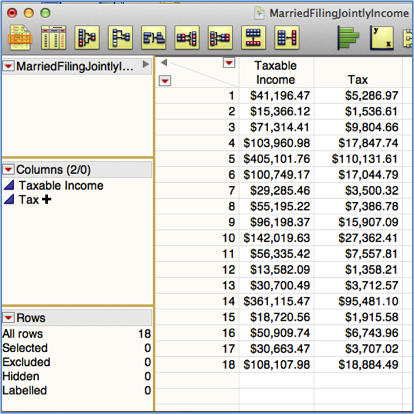For those of you who prefer scripting, the solution is similar. In the script below, I’ve written values directly to the table without a formula, but using the formula() option in the << New Column () message would work just as well.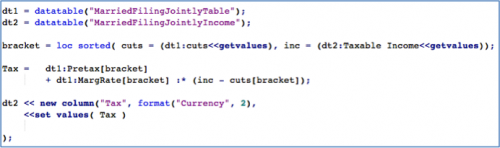And there you have it. That’s all there is to using lookup tables in JMP! If you’re like many of our customers, you’ll see plenty of opportunities for their use — enjoy.

Community Member

Walter Paczkowski wrote:

This is a great tutorial! I have to recode all the time so this will streamline the process. And the use of a script is good to know. Any restrictions on using scripts in formulas?

Like length?

Waltbrady_brady
Staff

Walt, I'm not aware of a length restriction, but will say that when you're using a more complex formula (for example, involving loops or dynamically assigned values) you will need to use some more advanced ideas (the eval() function comes to mind) to make sure the formula works properly.

Community Member

I've successfully used the Loc Sorted() script in formulas and am grateful to have come across it. However, is there something similar that can match text to text? I have data with a column containing many job titles. I've created a summary list of the unique titles and from that created a column of job "categories". Now I'd like to match the job titles in my master list with the categories I created. Any ideas?

Thank you in advance!

Bruce

Labels